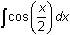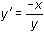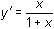Module 18 - Antiderivatives as Indefinite Integrals and Differential Equations Introduction | Lesson 1 | Lesson 2 | Lesson 3 | Lesson 4 | Self-Test Self Test Illustrate the family of graphs represented byand interpret the result. Use the deSolve( command to find the general solution to the differential equation y' = x3ex. Solve the initial value problem y' = y*(10 – y) and y(0) = 1. Don't forget the multiplication symbol between y and (10 – y). If y' = ln(x) and y(1) = 2, find y(3). Graph the slope field for the differential equationGraph the solution to the initial-value problemand y(0) = 1. Click here to check your answers. < Back ©Copyright 2007 All rights reserved. | Trademarks | Privacy Policy | Link Policy